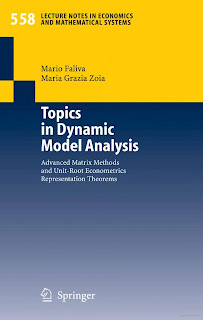MATRIX MATHEMATICS

# Topics in Dynamic Model Analysis Advanced Matrix Methods and Unit Root Econometrics Representation Theorems Free DownloadClassical econometrics – which plunges its roots in economic theory with simultaneous equations models (SEM) as offshoots – and time series econometrics – which stems from economic data with vector autoregr- sive (VAR) models as offsprings – scour, like the Janus’s facing heads, the flowing of economic variables so as to bring to the fore their autonomous and non-autonomous dynamics. It is up to the so-called final form of a dy namic SEM, on the one hand, and to the so-called representation theorems of (unit-root) VAR models, on the other, to provide informative closed form expressions for the trajectories, or time paths, of the economic vari ables of interest. Should we look at the issues just put forward from a mathematical standpoint, the emblematic models of both classical and time series econometrics would turn out to be difference equation systems with ad hoc characteristics, whose solutions are attained via a final form or a represen tation theorem approach.